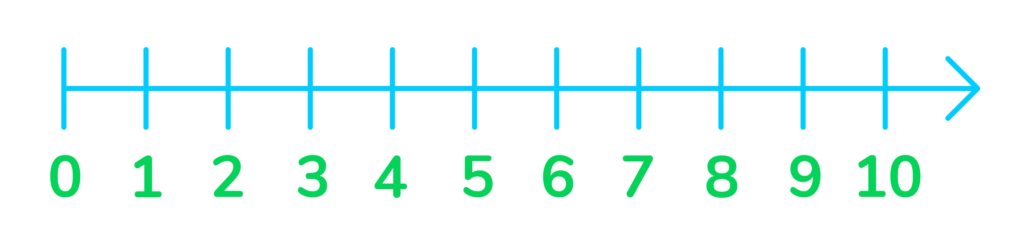# What Are Whole Numbers?

Whole numbers are a set of numbers that includes positive integers and 0. This article covers everything you need to know about whole numbers.Author
Katie WickliffPublished
October 20, 2023# What Are Whole Numbers?

Whole numbers are are a set of numbers that includes positive integers and 0. This article covers everything you need to know about whole numbers.Author
Katie WickliffPublished
Oct 20, 2023# What Are Whole Numbers?

Whole numbers are are a set of numbers that includes positive integers and 0. This article covers everything you need to know about whole numbers.Author
Katie WickliffPublished
Oct 20, 2023Key takeaways

• Whole numbers are not parts of numbers–such as decimals or fractions. They are also not negative numbers.
• Whole numbers, besides 0, are also called “counting numbers.”
• Several properties help us solve mathematical operations with whole numbers.

0, 65, 456, 23, 4,500, 72…

While this may seem like a random set of unrelated numbers, they all have something in common.

Do you know what it is?

Each number on this list is known as a whole number

## Whole Numbers DefinitionWhole numbers are all positive integers, beginning at zero and stretching to infinity. Decimals, fractions, and negative numbers are not whole numbers. All whole numbers, except for zero, are also called counting numbers or natural numbers.

## Whole Numbers on a Number LineWhole numbers can easily be represented on a number line. Whole numbers on a number line start at zero and increase by 1s at an equal distance from left to right along the line. Let’s look at the example below:Zero is the smallest whole number, and the black arrow shows us that the whole numbers stretch on infinitely.

## Natural Numbers vs Whole Numbers• The set of whole numbers begins with 0, while the set of natural numbers begins with 1.
• The smallest whole number is 0, and the smallest natural number is 1.
• Natural numbers are also called counting numbers. Not all whole numbers are called counting numbers because 0 is not considered a counting number.
• A set of natural numbers are represented by the letter “N,” while a set of whole numbers are represented by the letter “W.”## Properties of Whole NumbersFour properties help perform operations with whole numbers. These are:

1. Closure Property
2. Associative Property
3. Commutative Property
4. Distributive Property

Closure Property states that adding and multiplying two whole numbers will always have a whole number sum or product. It is impossible to add or multiply two whole numbers and get a negative number, fraction, or decimal answer.

Associative Property states that three whole numbers added or multiplied together will always have the same sum or product, no matter how the numbers are arranged. For example:

3+4+2=9

4+2+3=9

2+3+4=9

No matter how the 2, 3, and 4 are arranged, the sum will always be 9.

6x2x2=24

2x2x6=24

2x6x2=24

No matter how the 2, 2, and 6 are arranged, the product will always be 24

Commutive Property states that two whole numbers added or multiplied together will always have the same sum or product, no matter how the numbers are arranged. This is the same as the associative property, except with two whole numbers instead of three. For example:

4+2=6

2+4=6

No matter how the 4 and 2 are arranged, the sum will always be 6.

3×7=21

7×3=21

No matter how the 3 and 7 are arranged, the sum will always be 21.

Distributive Property states that in an expression such as A(B+C), you can distribute A to each of the addends (B and C) and multiply them then add the two products together: (AB)+ (AC). Here’s a whole numbers example of this property:

5x(2+3)=25

You can solve this by adding the 2+3 together first and then multiplying that answer by 5 to reach the product of 25.

However, if you use the distributive property, you’d solve like this:

5x(2+3)= 5×2 + 5×3= 25

So, whether you add the numbers in parenthesis before or after multiplying, the answer will be the same.

## Whole Number Practice Problems

92, 93, 94

True

There are 11 whole numbers between 33 and 45. They are: 34, 35, 36, 37, 38, 39, 40, 41, 42, 43, 44.

CNo, negative numbers are not whole numbers.

Positive integers are whole numbers. However, negative integers are not whole numbers.

Yes, 0 is a whole number.Lesson credits

Katie Wickliff

Katie holds a master’s degree in Education from the University of Colorado and a bachelor’s degree in both Journalism and English from The University of Iowa. She has over 15 years of education experience as a K-12 classroom teacher and Orton-Gillingham certified tutor. Most importantly, Katie is the mother of two elementary students, ages 8 and 11. She is passionate about math education and firmly believes that the right tools and support will help every student reach their full potential.

Katie Wickliff

Katie holds a master’s degree in Education from the University of Colorado and a bachelor’s degree in both Journalism and English from The University of Iowa. She has over 15 years of education experience as a K-12 classroom teacher and Orton-Gillingham certified tutor. Most importantly, Katie is the mother of two elementary students, ages 8 and 11. She is passionate about math education and firmly believes that the right tools and support will help every student reach their full potential.# Are you a parent, teacher or student?

Are you a parent or teacher?

## Hi there!

Book a chat with our team